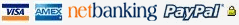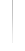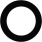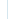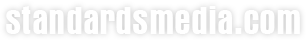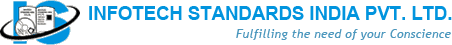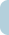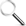Books Standards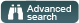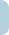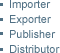Email Id: Password: Forgot Password? Not Registered Yet? REGISTER NOW
Welcome Guest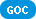|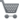0 items in your shopping cart

BROWSE BY SUBJECTSAuditingEnergyEnvironment EngineeringPollutionMechanical EngineeringSafetyElectrical EngineeringEngineeringProject ManagementManagementMetallurgyCivil EngineeringChemicalManufacturingTQMPowerPetroleumSix SigmaLeanWeldingDrilling5STPMDie CastingUDCPressure Vessel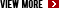BROWSE BY PUBLISHERS***

BROWSE BY STANDARDSAIAGAPIASMEASTMAWWABISBSICTIDINIECISONFPASAE

BROWSE BY CATEGORY***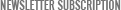Join our mailing list to recieve newsletters

Engineering Analysis : Interactive Methods and Programs with FORTRAN,Quick BASIC,MATLAB & Mathematic

Send to friend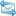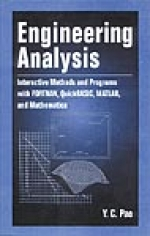Title: Engineering Analysis : Interactive Methods and Programs with FORTRAN,Quick BASIC,MATLAB & Mathematic Author: Y.C. Pao ISBN: 084932016X / 9780849320163Format: Hard CoverPages: 384Publisher: CRC PressYear: 1999Availability: In Stock Description Contents This book provides a concise introduction to numerical concepts in engineering analysis, using FORTRAN, QuickBASIC, MATLAB, and Mathematica to illustrate the examples. Discussions include : • Matrix algebra and analysis • Solution of matrix equations • Methods of curve fit • Methods for finding the roots of polynomials and transcendental equations • Finite differences and methods for interpolation and numerical differentiation • Numerical computation of single and double integrals • Numerical solution of ordinary differential equations Engineering Analysis : • Teaches readers to become proficient in FORTRAN or QuickBASIC programming to solve engineering problems • Provides an introduction to MATLAB and Mathematica, enabling readers to write supplementary m-files for MATLAB and toolkits for Mathematica using C-like commands The book emphasizes interactive operation in developing computer programs throughout, enabling the values of the parameters involved in the problem to be entered by the user of the program via a keyboard. In introducing each numerical method, Engineering Analysis gives minimum mathematical derivations but provides a thorough explanation of computational procedures to solve a specific problem. It serves as an exceptional text for self-study as well as resource for general reference. Preface and Acknowledgments Chapter 1 : Matrix Algebra and Solution of Matrix Equations Chapter 2 : Exact, Least-Squares, and Spline Curve-Fits Chapter 3 : Roots of Polynomial and Transcendental Equations Chapter 4 : Finite Differences, Interpolation, and Numerical Differentiation Chapter 5 : Numerical Integration and Program Volume Chapter 6 : Ordinary Differential Equations – Initial and Boundary Value Problems Chapter 7 : Eigenvalue and Eigenvector Problems Chapter 8 : Partial Differential Equations General Index FORTRAN Commands and Programs Index QuickBASIC Commands and Programs Index MATLAB Commands and Programs Index Mathematica Commands and Programs IndexAbout Us | Contact us